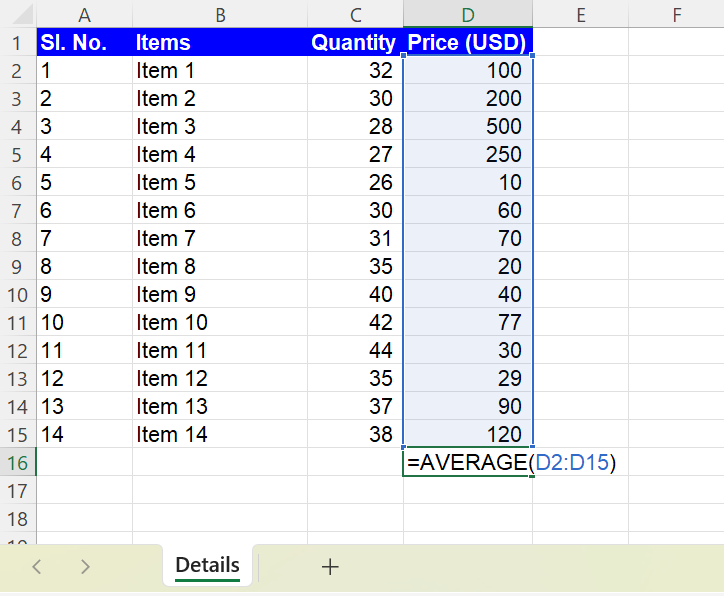Excel Formulas

# How to Use AVERAGE Function in Excel (Simple Guide)

In this article, you will learn how to calculate averages in Excel using the AVERAGE formula.

## Understanding the AVERAGE formula in Excel

The AVERAGE function in Excel is used to calculate the average of the selected values. In other words, it delivers the arithmetic mean of the data selected.

## The AVERAGE function syntax in Excel

``=AVERAGE(number1, [number2], ...)``

In the above syntax, the average of each number selected will be calculated.

For example: if 3 is number 1 and 6 is number 2, the formula =AVERAGE(3,6) will calculate the average value to be 4.5.

## When to use the AVERAGE formula in Excel

Here are some common scenarios where you might want to use the AVERAGE function:

Analyzing data: If you have a set of data that you want to analyze, you can use the AVERAGE formula to calculate the average value of the data. For example, if you have a price list of items sold, you can use the AVERAGE formula in Excel to calculate the average price.

Budgeting: If you are creating a budget or financial plan, you may want to use the AVERAGE function in Excel to calculate the average monthly income or expenses.

Forecasting: If you are trying to predict future trends based on past performance, you may want to use the AVERAGE formula to calculate the average growth rate or other statistical measures.

Evaluating performance: If you are evaluating the performance of a sales team or other group, you can use the AVERAGE function in Excel to calculate the average sales figures or other metrics.

Overall, the AVERAGE formula is a useful tool for a wide range of applications, allowing you to quickly calculate the average value of a set of numbers in Excel.

## Inserting the AVERAGE function in Excel

Step 1: Type “=AVERAGE” in the cell where you want to calculate the mean

Step 2: Once the formula prompt opens up, click on the AVERAGE formula

Step 3: Select the values one by one by separating them with “,” (commas). You can also select an array of data as shown in the image below.Image showing the AVERAGE formula used to select an array of data in Excel

TIP: The AVERAGE function in Excel recognizes ‘0’ (zero) and includes it while calculating the average. Hence, if any data point is missing, avoid denoting it as zero. Instead, you can denote the missing value with free text such as ‘missing’ or ‘not available’ or any other text comment and Excel will exclude it while calculating the mean.

## Analyze your live financial data in a snap in Google Sheets

Are you learning this formula to visualize financial data, build a financial model, or conduct financial analysis? In that case, LiveFlow may help you automate manual workflows, update numbers in real-time, and save time. You can access various financial templates on our website, from the simple Income Statement to Multi-Currency Consolidated Financial Statement. Are you interested in this product but are an Excel user? That’s not a problem at all. You can connect Google Sheets to Excel quickly.

You can learn about other Excel and Google Sheets formulas and tips that are not mentioned here on this page: LiveFlow‘s How to Guides

Learn how to do this step-by-step in the video below 👇

## Do you need personal help?

Our team of real people are here to help you any time between 9am and 10pm EST.Email us at: help@liveflow.ioCall us at +1 (415) 650-1711